# How to Calculate and Solve for the Width, Height, Length and Area of a Rectangular Prism | The Calculator Encyclopedia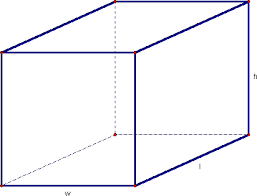The image above is a rectangular prism.

To compute the area of a rectangular prism requires three essential parameters which are the length, width and height of the rectangular prism.

A = 2wl + 2hl + 2hw

Where;
A = Area of the rectangular prism
l = Length of the rectangular prism
w = Width of the rectangular prism
h = Height of the rectangular prism

Let’s solve an example;
Find the area of a rectangular prism with a width of 10 cm, a height of 17 cm and a length of 14 cm.

This implies that;
l = Length of the rectangular prism = 14 cm
w = Width of the rectangular prism = 10 cm
h = Height of the rectangular prism = 17 cm

A = 2wl + 2hl + 2hw
A = 2 x 10 x 14 + 2 x 17 x 14 + 2 x 17 x 10
A = 280 + 476 + 340
A = 1096

Therefore, the area of the rectangular prism is 1096 cm2.

Calculating the Length of a Rectangular Prism using the Area, Width and Height of the Rectangular Prism.

l = A – 2hw / 2 (w + h)

Where;
l = Length of the rectangular prism
A = Area of the rectangular prism
w = Width of the rectangular prism
h = Height of the rectangular prism

Let’s solve an example;
Find the length of a rectangular prism with a width of 9 cm, a height of 12 cm and a Area of 250 cm2.

This implies that;
w = Width of the rectangular prism = 9 cm
h = Height of the rectangular prism = 12 cm
A = Area of the rectangular prism = 250 cm2

l = A – 2hw / 2 (w + h)
l = 250 – 2 x 12 x 9 / 2 (9 + 12)
l = 250 – 216 / 2 (21)
l = 34 / 42
l = 0.81

Therefore, the length of the rectangular prism is 0.81 cm.

Calculating the Width of a Rectangular Prism using the Area, Length and Height of the Rectangular Prism.

w = A – 2hl / 2 (l + h)

Where;
l = Length of the rectangular prism
A = Area of the rectangular prism
w = Width of the rectangular prism
h = Height of the rectangular prism

Let’s solve an example;
Find the width of a rectangular prism with a length of  8 cm, a height of 11 cm and a Area of 340 cm2.

This implies that;
l = Length of the rectangular prism = 8 cm
h = Height of the rectangular prism = 11 cm
A = Area of the rectangular prism = 340 cm2

w = A – 2hl / 2 (l + h)
w = 340 – 2 x 11 x 8 / 2 (8 + 11)
w = 340 – 176 / 2 (19)
w = 164 / 38
w = 4.32

Therefore, the width of the rectangular prism is 4.32 cm.

Calculating the Height of a Rectangular Prism using the Area, Length and Width of the Rectangular Prism.

h = A – 2wl / 2 (l + w)

Where;
l = Length of the rectangular prism
A = Area of the rectangular prism
w = Width of the rectangular prism
h = Height of the rectangular prism

Let’s solve an example;
Find the height of a rectangular prism with a width of  7 cm, a length of 13 cm and a Area of 400 cm2.

This implies that;
l = Length of the rectangular prism = 13 cm
w = Width of the rectangular prism = 7 cm
A = Area of the rectangular prism = 400 cm2

h = A – 2wl / 2 (l + w)
h = 400 – 2 x 7 x 13 / 2 (13 + 7)
h = 400 – 182 / 2 (20)
h = 218 / 40
h = 5.45

Therefore, the height of the rectangular prism is 5.45 cm.

Nickzom Calculator – The Calculator Encyclopedia is capable of calculating the area of a rectangular prism.

To get the answer and workings of the area of a rectangular prism using the Nickzom Calculator – The Calculator Encyclopedia. First, you need to obtain the app.

You can get this app via any of these means:

To get access to the professional version via web, you need to register and subscribe for NGN 1,500 per annum to have utter access to all functionalities.
You can also try the demo version via https://www.nickzom.org/calculator

Once, you have obtained the calculator encyclopedia app, proceed to the Calculator Map, then click on Mensuration under the Mathematics section.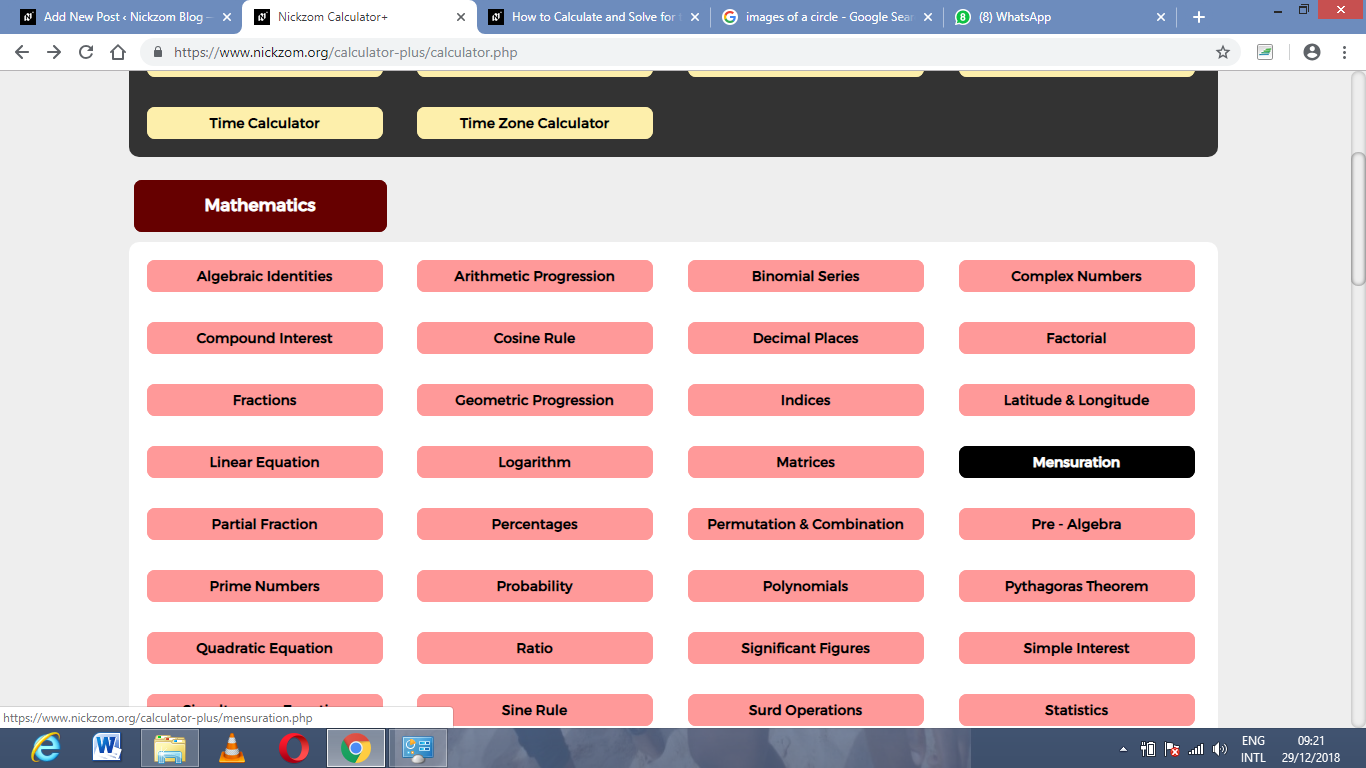Now, click on Area of a rectangular prism under MensurationThe screenshot below displays the page or activity to enter your values, to get the answer for the area of a rectangular prism according to the respective parameters which are the length, the width and the height of the rectangular prism.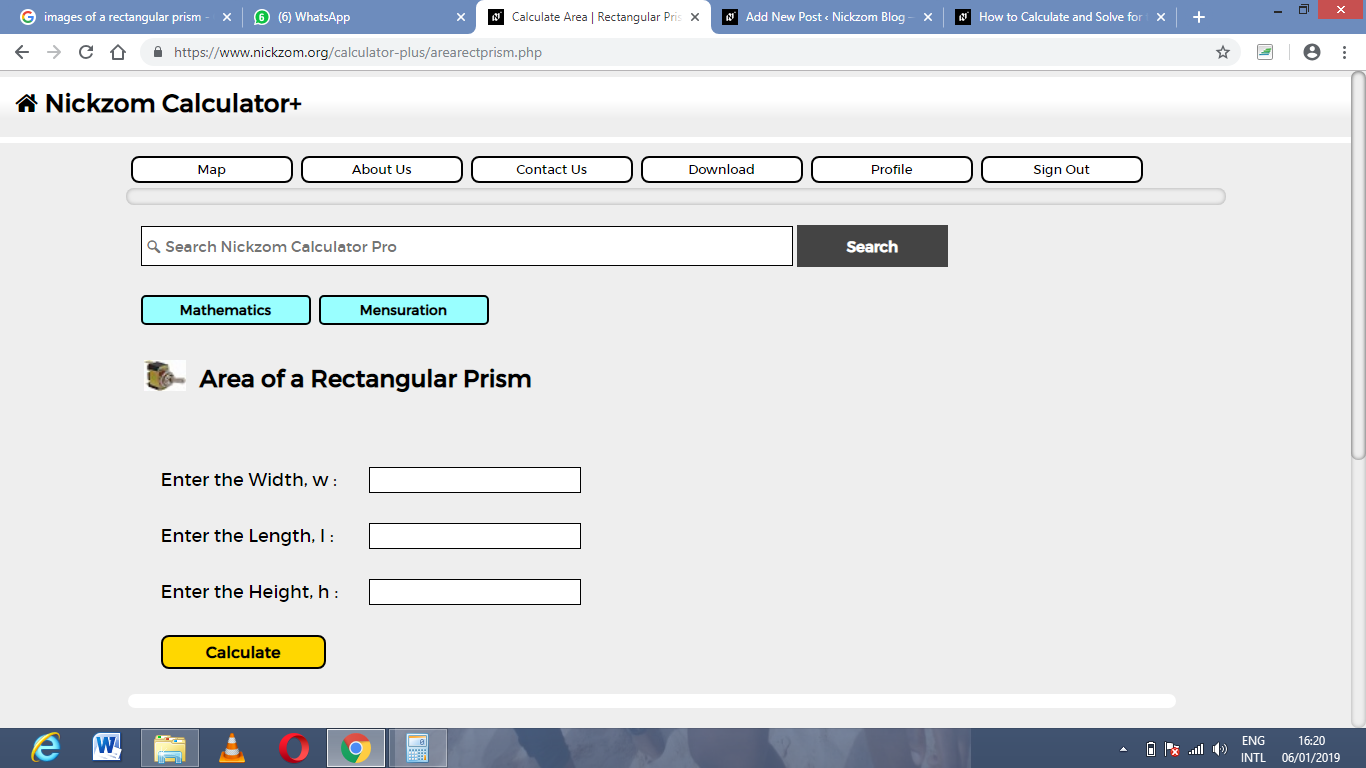Now, enter the values appropriately and accordingly for the parameters as required by the example above where the width is 10 cm, the length is 14 cm and the height is 17 cm.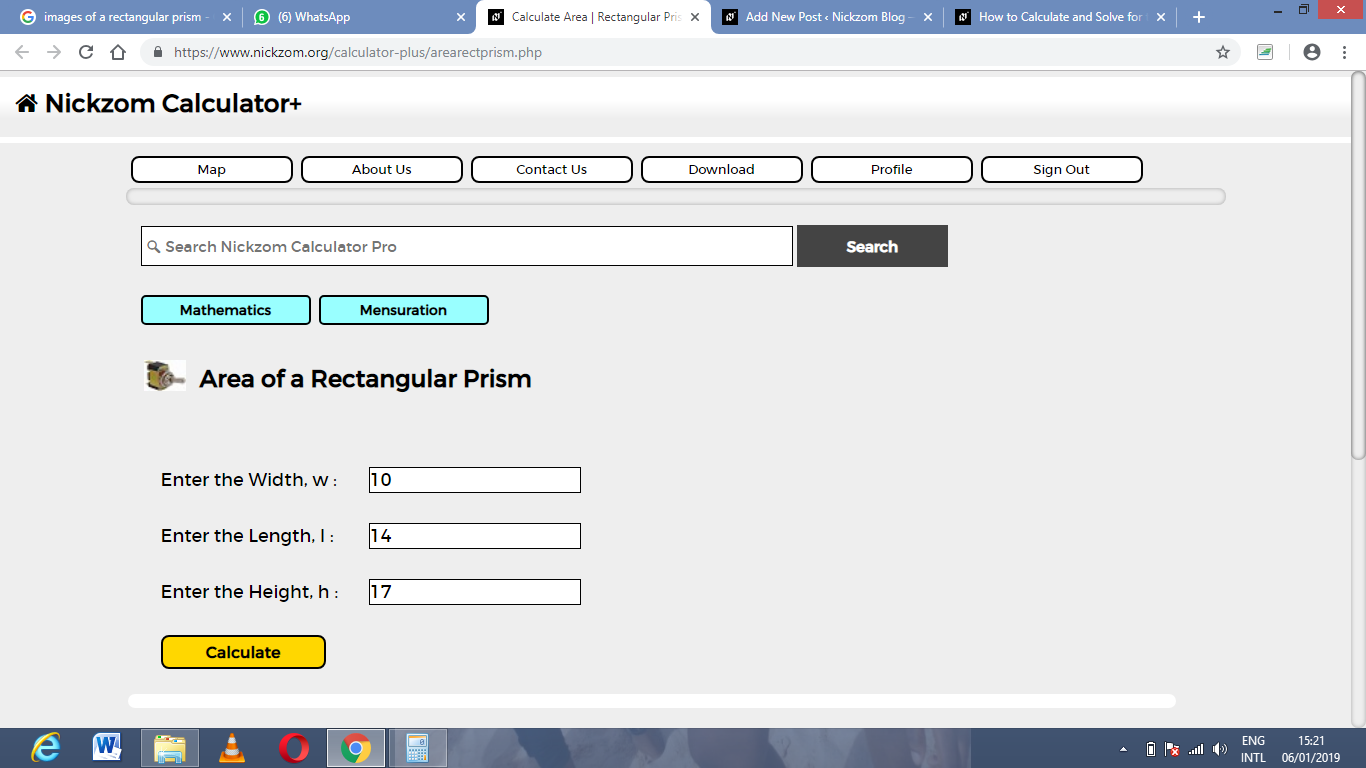Finally, Click on Calculate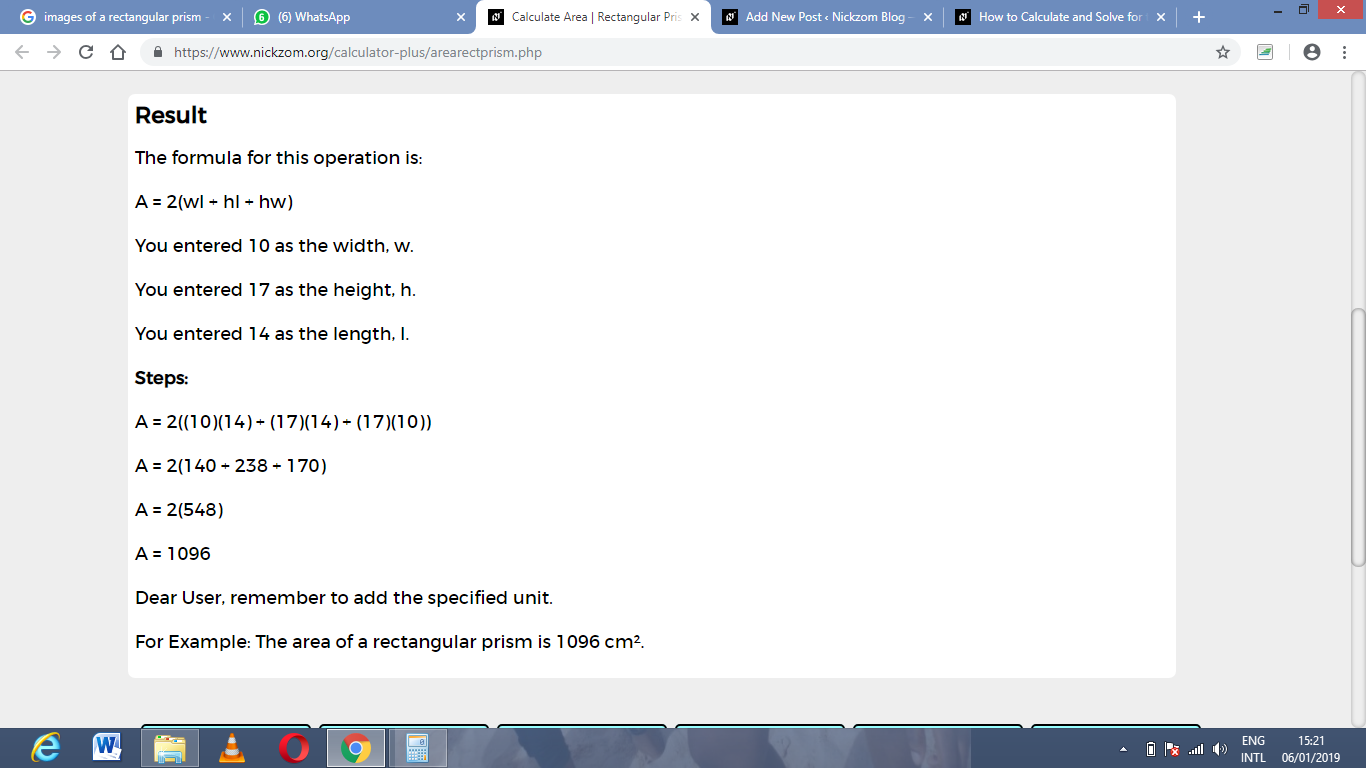As you can see from the screenshot above, Nickzom Calculator – The Calculator Encyclopedia solves for the area of a rectangular prism and presents the formula, workings and steps too.